# Introduction

Proof of Capacity (PoC) is a sound and fair consensus algorithm, field tested for more than 5 years now in the Burstcoin network. Sound because it can use off-the-shelf equipment and is energy efficient. Fair because it has a very low entry barrier and shows a more linear scaling.

Essentially, PoC consists of storing hard to compute hashes and then reusing these hashes every time a block is forged. The basic idea is, the more capacity you have, the more hashes you can store, the higher your commitment is to the system and the higher should be your reward (your chances to forge a block).

The current implementation in Burstcoin, the pioneer of Proof of Capacity, results in very good block time averages, with daily deviations smaller than 5% around the target value of 4 minutes (240 seconds). The individual block times, on the other hand, show a broad deviation with block times typically ranging from 10 seconds to 2’000 seconds (30 minutes). This is also the case for the many Burstcoin copycat coins (which I will not list here). In this article, this issue is discussed and a simple modification to reduce the individual block times variation is proposed.

# Burstcoin Average Block Time

Burstcoin contains a block time control algorithm that aims for 4 minute blocks (on average). It is a feedback control algorithm acting on every new block (based on past block times) with heuristic rules to avoid instabilities. So the so called difficulty is adjusted to absorb any network changes (miners coming and going). This method is very efficient and produces averages very close to 4 minutes: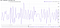Burstcoin daily average block times for a timespan of 3 months (source https://explorer.burstcoin.network/).

As can be seen, the block time control algorithm is very robust. It keeps the average block time very near to the 4 minutes (240 seconds) target, deviating no more than 10 seconds from the average, which means less than 5 % deviation.

The efficiency of the block time control algorithm is even more clear if we compare Burstcoin average block times with those from Bitcoin for the same time period (having a target average of 10 minutes):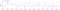Bitcoin daily average block times for a timespan of 3 months (source https://bitinfocharts.com/).

As can be seen on the above chart, typical Bitcoin average block times can deviate as much as 2 minutes or 20 % from the target.

# Individual Block Times

Although the Burstcoin network has shown exceptional average block times, with a deviation usually less than 5 % from the target value of 4 minutes, it currently shows a high deviation on individual block times.

Each day, around 360 new blocks are added to the Burstcoin chain. If we plot the block time of every block for a typical day, we end up with a chart very similar to the following (source code):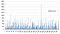Typical block times (in seconds) for an entire day on the Burstcoin chain.

As discussed before, a typical day has an average block time of 240 seconds, but as can be seen in the above chart, there is a broad deviation for individual values. A very similar chart is observed every day and the same is true if we use data from the Burstcoin testnet (but with an even higher deviation due to the smaller network size).

This high deviation for individual values is even more clear if we sort the block times in increasing order (using the very same data):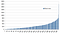Typical block times (in seconds) for an entire day on the Burstcoin chain, sorted in increasing order.

In the chart above with the block time data sorted, it is more clear that the distribution is not symmetric around the average. We can see that very short blocks are often found (several around 10 seconds and a lot below 100 seconds) and, on the other hand, a few very long blocks are present (almost 2’000 seconds). The average, nonetheless, is always very close to 240 seconds due to the base target control (or difficulty control), as previously discussed.

The first question would be, what is the source of this problem? For sure it is not the block time control (base target or difficulty) algorithm, since it is working perfectly on the daily basis even with very diverse individual block times. Also the base target typically varies only by 50 % during one day, which alone cannot explain the variation from 10 to 2’000 seconds.

The second question would be, is this phenomenon unique to Proof of Capacity algorithms, or is it also observed on regular Proof of Work (PoW) chains like Bitcoin? The key point here is the following: in PoW algorithms every second a block is late is an additional second to compute more hashes (use more nonces) and increase the chance to solve the block. So, there is this additional control mechanism to avoid very long blocks, which is currently unavailable for PoC. In PoC all your hashes (or nonces) are already in place (stored on your hard disk), regardless of the block time. Thus, there is no action available in the event no one in the network has a good hash (with a short deadline).

So, in order to get more stable individual block times, an additional mechanism could be added to avoid both very short deadlines and very long blocks. It is important to notice that both short and long block times are tied together by the average block time control. If any mechanism tampers with the distribution average, the block time control will act, changing the difficulty to keep the average close to 240 seconds.

# Theoretical Analysis

(Readers not interested in a more detailed analysis or not used to integral calculus can simply skip this section.)

In the study of continuous-time stochastic processes, the exponential distribution can be used to model the time until something happens in the process. It is defined by:

where λ > 0 is known as the rate of the distribution.

A very interesting property of this distribution is that its expected (average) value is simply 1/λ:

Thus, if one wants to model our block time distribution with this function, λ must be equal to 1/240.

Another interesting property of the exponential distribution function, applied to our case, is that the probability of a block taking longer than t is given by:

By using this result, the exponential distribution applied to the Burstcoin block time (λ=1/240) is as follows: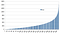Block time distribution (in seconds) calculated assuming an exponential distribution with an average value of 240.

As can be seen, this theoretical curve is very similar to the empirically observed one. Again, this indicates that the block time distribution observed is a natural response of the PoC algorithm and its stochastic nature, not coming from the base target (or difficulty) algorithm.

As discussed before, when we compare PoC and PoW, the former has this additional block time control mechanism absent. This makes individual block times vary enormously (typically from 10 seconds up to 2’000 seconds every day).

Currently, the deadline is computed as follows (Burstkit4J source code):

where hit is a number derived from the account id, nonce, etc. and baseTarget represents the current difficulty (which is controlled to keep the average close to 240 seconds).

This simple deadline computation was inherited from PoW chains like Bitcoin. In regular PoW implementations, every second a new set of hashes are computed. If snapshots were taken over time, selecting only the current best hash among all miners, they would also show a very large deviation (exponential distribution). However, as the best hash over the entire block time is the one solving the block, the result is reasonably stable with that simple deadline formula. However, this same simple computation leads to high block time deviations on individual values with PoC algorithms, since you are not allowed to explore new nonces as the block time passes. Thus, a possible improvement for PoC algorithms would be to use a function nonlinear on hit. This function needs to be monotonic so all the other principles of the consensus algorithm are kept unaffected. Preferably, it also needs to produce a similar average, so the average block time control does not suffer during the hard-fork event. One simple option would be to compute the deadline as follows:

By using the square root, all deadlines become smaller, but in a nonlinear way. This makes larger individual values closer to each other and leads to an average of around 14 (instead of 240). If the average is smaller, the block time control would adjust the base target to take back the average value to 240. But, in the event of a switch (hard-fork), block times would be very inconsistent until adjusted. So, in order to make the switch as smooth as possible, we recover an average value around 240 by multiplying the square root result by a factor of 16. This number was obtained empirically with data from mainnet for several different days but it is very close to 240/sqrt(240) = 15.49.

A typical result with the nonlinear deadline computation proposed above is the following: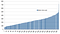Typical block times (in seconds) for an entire day on the Burstcoin chain assuming the sqrt function for deadline computation.

As can be seen, with the square root version, the block times previously ranging from 10 to 2’000 seconds will range from 50 to 720 seconds (1 to 12 minutes).

This modification would require a hard-fork. All nodes and pool software would need to be updated. Depending on how node and pool software are updated, mining software could be kept as they currently are.

Other nonlinear functions could be used, for instance the natural logarithm:

Again the factor of 45 was obtained empirically, but it is very close to 240/ln(240) = 43.79. By using this logarithm version, the individual block time values would be even closer to the average value, ranging typically from 100 to 360 seconds (1.6 to 6 minutes):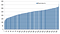Typical block times (in seconds) for an entire day on the Burstcoin chain assuming the logarithm function for deadline computation.

As can be seen in the above chart, the response becomes more linear when the logarithm transformation is applied (since logarithm is the inverse of exponential — see the Theoretical Analysis section for rationale on this). Taking the case of the logarithm function, even more interesting is the fact that deadlines of 10 and 11 seconds in the original method (apart by only 1 second of each other and a probable cause of temporary chain splits) become 105 and 115 seconds (respectively) when computed with the logarithmic function.

# Conclusions

Proof of Capacity (PoC) implemented in Burstcoin is the living proof that something better than Proof of Work (PoW) exists, now running since 2014. It not only avoids the waste of huge amounts of energy but also provides more stable average block times. However, as currently implemented, individual block times vary enormously. This is due to a missing control, lost by the nature of PoC (no additional computations during the mining process). However, this problem can be greatly reduced by using a simple nonlinear function for the deadline computations. If a square root function is used, block times would vary typically from 50 to 720 seconds, keeping the average of 240 seconds. If a natural logarithm function is used, block times would be typically in the range of 100 to 360 seconds.

## jjos

Energy efficiency enthusiast interested in encryption…

Energy efficiency enthusiast interested in encryption algorithms and blockchain. Has developed virtual machines and the BlockTalk platform, allowing to write smart contracts in Java.

Written by

## jj os

Energy efficiency enthusiast interested in encryption and blockchain. Has developed the BlockTalk platform, allowing to write smart contracts in Java.## jjos

Energy efficiency enthusiast interested in encryption algorithms and blockchain. Has developed virtual machines and the BlockTalk platform, allowing to write smart contracts in Java.

## More From Medium

Medium is an open platform where 170 million readers come to find insightful and dynamic thinking. Here, expert and undiscovered voices alike dive into the heart of any topic and bring new ideas to the surface. Learn more

Follow the writers, publications, and topics that matter to you, and you’ll see them on your homepage and in your inbox. Explore

If you have a story to tell, knowledge to share, or a perspective to offer — welcome home. It’s easy and free to post your thinking on any topic. Write on Medium